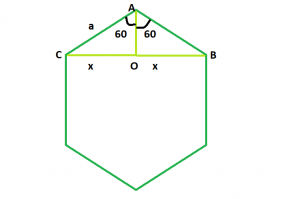GeeksforGeeks App
Open AppBrowser
Continue

# Diagonal of a Regular Hexagon

Given an integer a which is the side of a regular hexagon, the task is to find and print the length of its diagonal.
Examples:

Input: a = 6
Output: 10.38
Input: a = 9
Output: 15.57Approach: We know that the sum of interior angles of a polygon = (n – 2) * 180 where, n is the number of sides of the polygon.
So, sum of interior angles of a hexagon = 4 * 180 = 720 and each interior angle will be 120
Now, we have to find BC = 2 * x. If we draw a perpendicular AO on BC, we will see that the perpendicular bisects BC in BO and OC, as triangles AOB and AOC are congruent to each other.
So, in triangle AOB, sin(60) = x / a i.e. x = 0.866 * a
Therefore, diagonal length will be 2 * x i.e. 1.73 * a.
Below is the implementation of the above approach:

## C++

 `// C++ Program to find the diagonal``// of a regular hexagon``#include ``using` `namespace` `std;` `// Function to find the diagonal``// of a regular hexagon``float` `hexDiagonal(``float` `a)``{` `    ``// Side cannot be negative``    ``if` `(a < 0)``        ``return` `-1;` `    ``// Length of the diagonal``    ``float` `d = 1.73 * a;``    ``return` `d;``}` `// Driver code``int` `main()``{``    ``float` `a = 9;``    ``cout << hexDiagonal(a) << endl;``    ``return` `0;``}`

## Java

 `// Java Program to find the diagonal``// of a regular hexagon` `public` `class` `GFG``{` `    ``// Function to find the diagonal``    ``// of a regular hexagon``    ``static` `double` `hexDiagonal(``float` `a)``    ``{``    ` `        ``// Side cannot be negative``        ``if` `(a < ``0``)``            ``return` `-``1``;``    ` `        ``// Length of the diagonal``        ``double` `d = (``double``)``1.73` `* a;``        ``return` `d;``    ``}``    ` `    ``// Driver code``    ``public` `static` `void` `main(String []args)``    ``{``        ``float` `a = ``9``;``        ``System.out.println(hexDiagonal(a)) ;``    ``}``    ``// This code is contributed by Ryuga``}`

## Python3

 `# Python3 Program to find the diagonal``# of a regular hexagon` `# Function to find the diagonal``# of a regular hexagon``def` `hexDiagonal(a):` `    ``# Side cannot be negative``    ``if` `(a < ``0``):``        ``return` `-``1``;` `    ``# Length of the diagonal``    ``d ``=` `1.73` `*` `a;``    ``return` `d;`  `# Driver code``a ``=` `9``;``print``(hexDiagonal(a));` `# This code is contributed``# by Akanksha Rai`

## C#

 `// C# Program to find the diagonal ``// of a regular hexagon``using` `System ;``public` `class` `GFG``{``  ` `    ``// Function to find the diagonal ``    ``// of a regular hexagon ``    ``static` `double` `hexDiagonal(``float` `a) ``    ``{ ``      ` `        ``// Side cannot be negative ``        ``if` `(a < 0) ``            ``return` `-1; ``      ` `        ``// Length of the diagonal ``        ``double` `d = (``double``)1.73 * a; ``        ``return` `d; ``    ``} ``      ` `    ``// Driver code ``    ``public` `static` `void` `Main()``    ``{ ``        ``float` `a = 9; ``        ``Console.WriteLine(hexDiagonal(a)) ; ``    ``} ``    ``// This code is contributed by Subhadeep``}`

## PHP

 ``

## Javascript

 ``

Output:

`15.57`

Time Complexity: O(1)

Auxiliary Space: O(1)

My Personal Notes arrow_drop_up